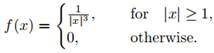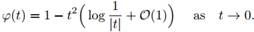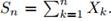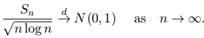### Create an Account

Home / Questions / There exist central limit theorems in spite of the fact that the variance is infinite Cons...

# There exist central limit theorems in spite of the fact that the variance is infinite Consider the two-sided Pareto distribution with density

There exist central limit theorems in spite of the fact that the variance is infinite: Consider the two-sided Pareto distribution with density(a) Prove that the characteristic function(b) Let X1, X2, ... be independent, identically distributed random variables with common density as above, and setProve thatApr 25 2020 View more View LessSubscribe To Get Solution